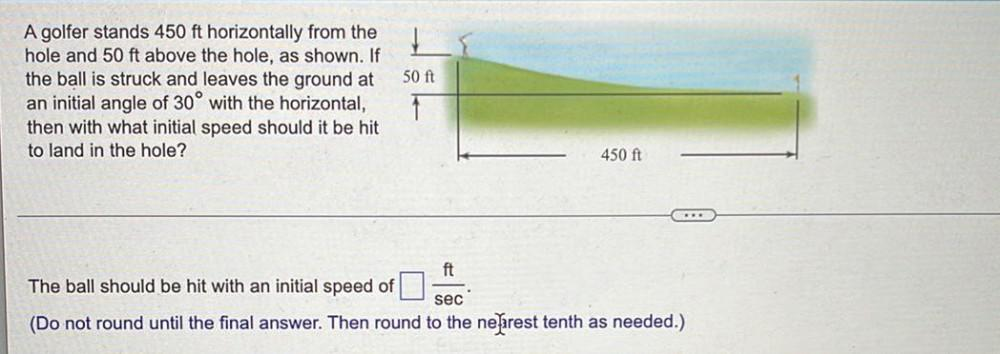Question:

# A golfer stands 450 ft horizontally from the hole and 50 ft above the hole, as shown. If the ball is struck and leaves the ground at an initial angle of 30° with the horizontal, then with what initialA golfer stands 450 ft horizontally from the hole and 50 ft above the hole, as shown. If the ball is struck and leaves the ground at an initial angle of 30° with the horizontal, then with what initial speed should it be hit to land in the hole? The ball should be hit with an initial speed of ___ ft/sec. (Do not round until the final answer. Then round to the nearest tenth as needed.)